# RC Circuit Formula Derivation Using Calculus

Eugene is a qualified control/instrumentation engineer Bsc (Eng) and has worked as a developer of electronics & software for SCADA systems.

## What Are Capacitors Used For?

Capacitors are used in electrical and electronic circuitry for a variety of reasons. Typically these are:

• Smoothing of rectified AC, pre-regulation in DC power supplies
• Setting the frequency of oscillators
• Bandwidth setting in low pass, high pass, band pass and band reject filters
• AC coupling in multistage amplifiers
• Bypassing transient currents on power supply lines to ICs (decoupling capacitors)
• Starting of induction motors
• EMI (Electromagnetic Interference) suppressors in electronic devices and electrical appliances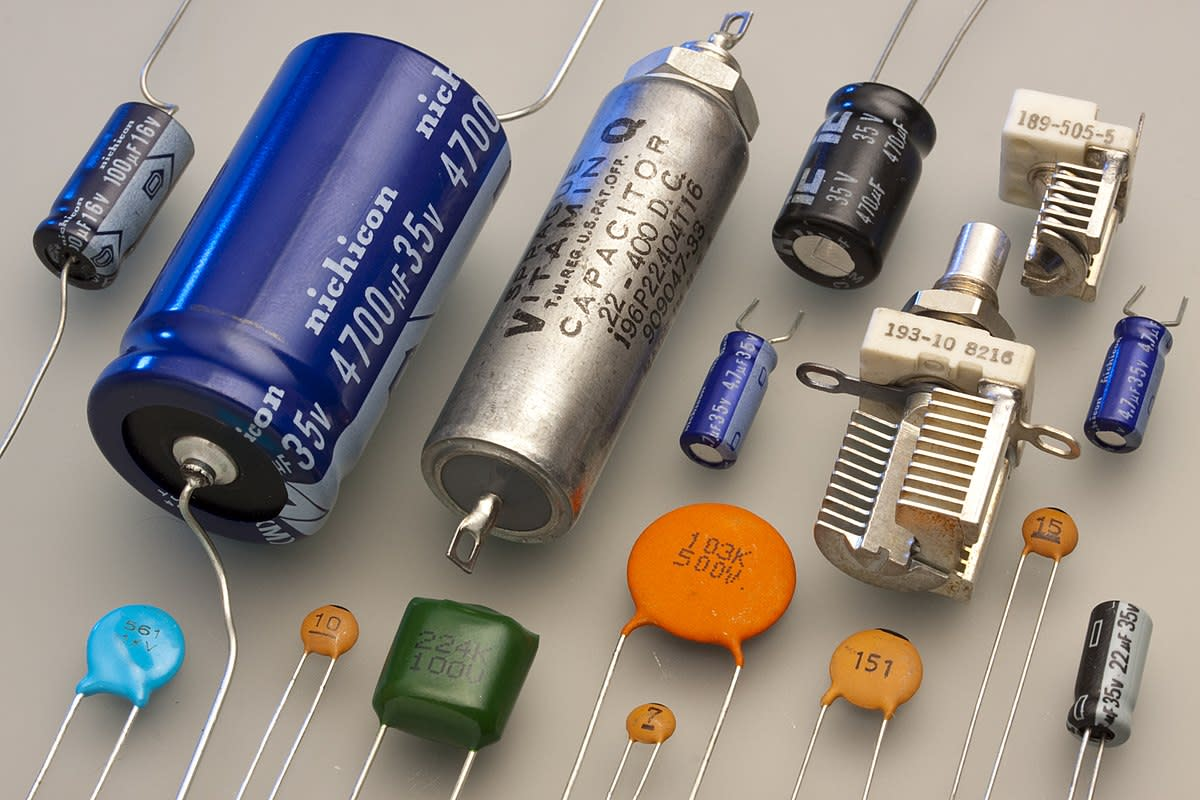Various type of capacitors used in electronicsEric Schrader, CC BY SA 2.0 via Wikimedia Commons

## Time Delays in Electronic Circuits

Whenever capacitance and resistance occur in an electronic or electrical circuit, the combination of these two quantities results in time delays in the transmission of signals. Sometimes this is the desired effect; other times it may be an unwanted side effect. Capacitance may be due to an electronic component, i.e a real physical capacitor, or stray capacitance caused by conductors in proximity (e.g. tracks on a circuit board or cores in a cable). Similarly, resistance may be the result of actual physical resistors or inherent series resistance of cables and components.

## Transient Response of an RC Circuit

In the circuit below, the switch is initially open, so before time t = 0, there is no voltage feeding the circuit. Once the switch closes, the supply voltage Vs is applied indefinitely. This is known as a step input. The response of the RC circuit is called a transient response, or step response for a step input.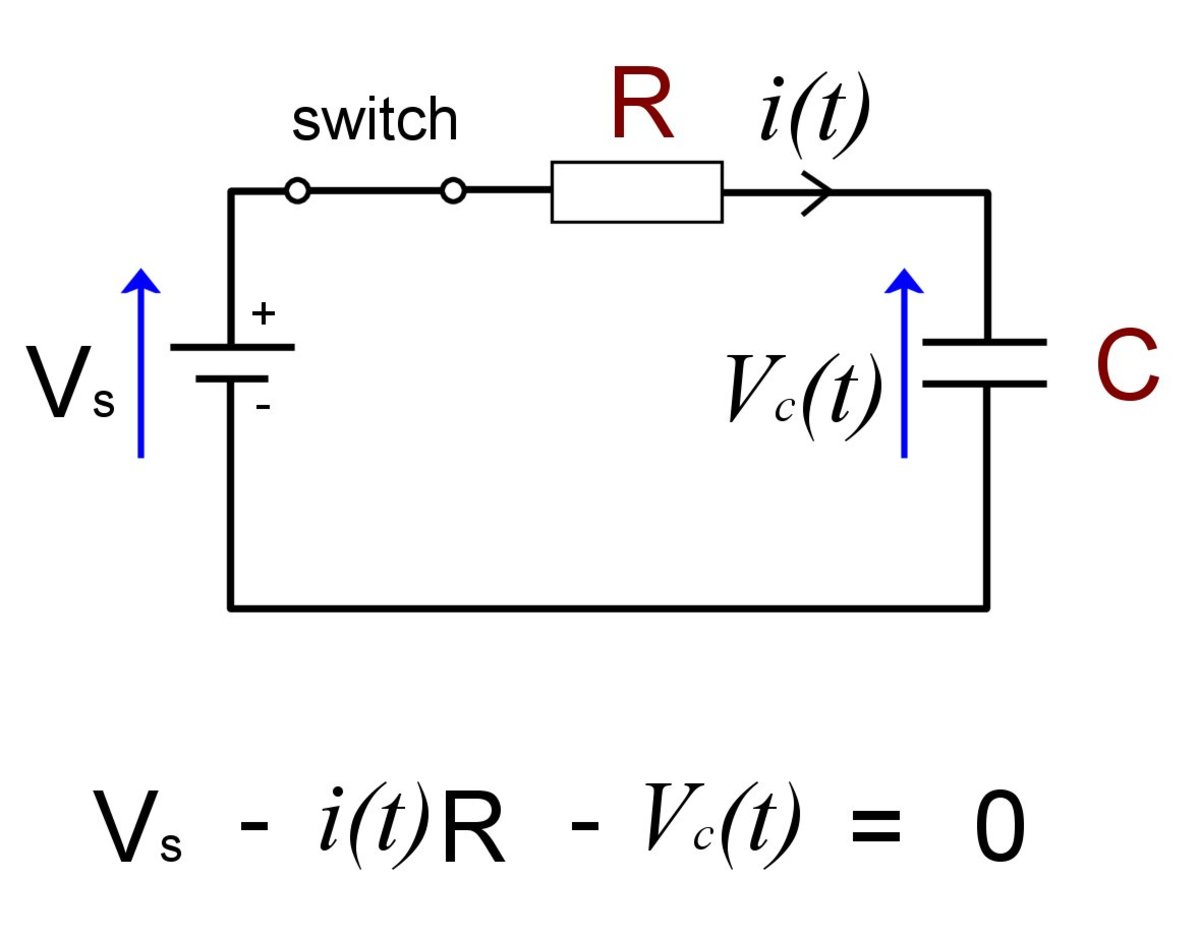Kirchoff's voltage law around an RC circuit.© Eugene Brennan

## Time Constant of an RC Circuit

When a step voltage is first applied to an RC circuit, the output voltage of the circuit doesn't change instantly. It has a time constant due to the fact that current needs to charge the capacitance. The time taken for the output voltage (the voltage on the capacitor) to reach 63% of its final value is known as the time constant, often represented by the Greek letter tau (τ). The time constant = RC, where R is the resistance in ohms and C is the capacitance in farads.

## Stages in the Charging of the Capacitor in an RC Circuit

In the circuit above, Vs is a DC voltage source. Once the switch closes, current starts to flow via the resistor R. Current begins to charge the capacitor and voltage across the capacitor Vc(t) starts to rise. Both Vc(t) and the current i(t) are functions of time.

Using Kirchhoff's voltage law around the circuit gives us an equation:

Vs - i(t)R - Vc(t) = 0 ............Eqn(1)

Initial Conditions:

If the capacitance of a capacitor in farads is C, the charge on the capacitor in coulombs is Q and the voltage across it is V, then:

C = Q/V

therefore

V = Q/C ..............................Eqn (2)

Scroll to Continue

## Read More From Owlcation

Since there is initially no charge Q on the capacitor C, the initial voltage Vc(t) is

Vc(0) = Q/C = 0/C = 0

The capacitor behaves initially like a short circuit and current is limited only by the series connected resistor R.

We check this by examining KVL for the circuit again:

Vs - i(t)R - Vc(t) = 0

Vc(t) at time t = 0 is Vc(0) = 0 so substituting into Eqn (1) for Vc(t) gives us:

Vs - i(0)R - 0 = 0

so Vs - i(0)R = 0

and rearranging Vs = i(0)R

therefore i(0) = Vs/R ...................Eqn (3)

So the initial conditions of the circuit are time t = 0, Q = 0, i(0) = Vs/R and Vc(0) = 0

Current through the resistor as the capacitor charges

As the capacitor charges, voltage across it increases since V = Q/C and Q is increasing. Let's look at what happens current.

Examining KVL for the circuit we know Vs - i(t)R - Vc(t) = 0

Rearranging the equation gives us the current through the resistor:

i(t) = ( Vs - Vc(t) ) / R

Vs and R are constants, so as the capacitor voltage Vc(t) increases , i(t) decreases from its initial value Vs/R at t = 0.
Since R and C are in series, i(t) is also the current through the capacitor.

Voltage across the capacitor as it charges

Again KVL tells us that Vs - i(t)R - Vc(t) = 0

Rearranging the equation gives us the capacitor voltage:

Vc(t) = Vs - i(t)R

Initially Vc(t) is 0, however as current decreases, the voltage dropped across the resistor R decreases and Vc(t) increases. After 4 time constants, it has reached 98% of its final value. After 5 times constants, i.e 5τ = 5RC, for all practical purposes, i(t) has decreased to 0 and Vc(t) = Vs - 0R = Vs.
So the capacitor voltage equals the supply voltage Vs.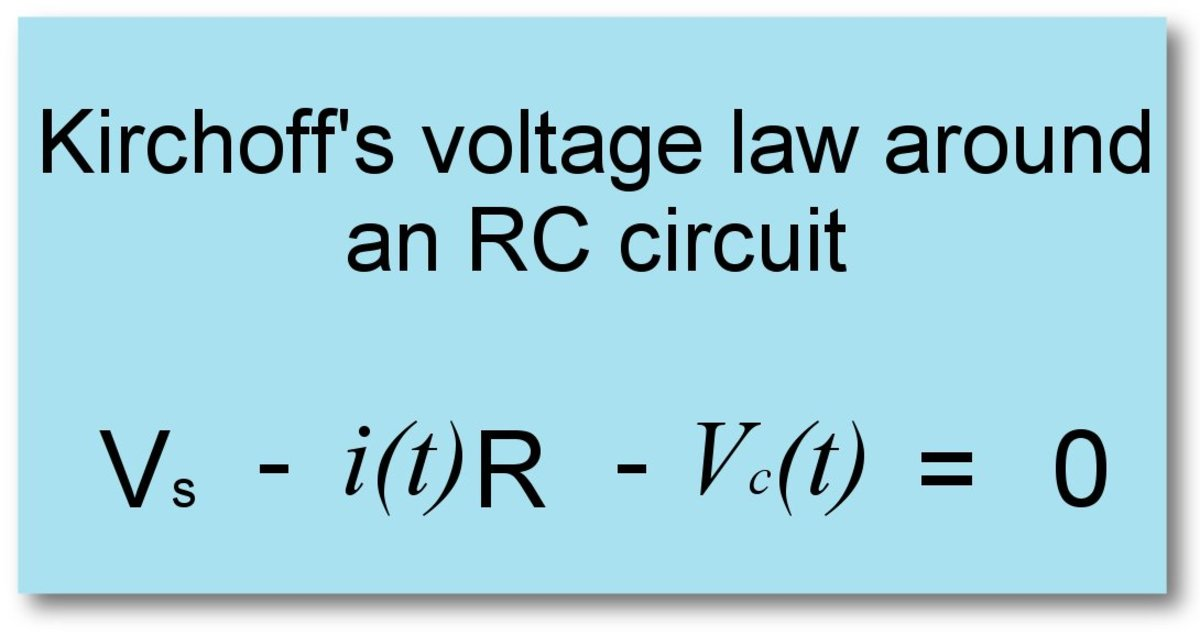Kirchoff's voltage law applied around an RC circuit© Eugene Brennan

## Transient Analysis of An RC Circuit

### Working Out an Equation for the Voltage Across the Capacitor in an RC Circuit

Working out the response of a circuit to an input that puts it in an unsteady state is known as transient analysis. Determining an expression for the voltage across the capacitor as a function of time (and also current through the resistor) requires some basic calculus.

### Analysis Part 1 - Working Out the Differential Equation for the Circuit:

From KVL we know that:

Vs - i(t)R - Vc(t) = 0

From Eqn (2) we know that for the capacitor C:

Vc = Q/C

Multiplying both sides of the equation by C and rearranging gives us:

Q = CVc

If we now take the derivative of both sides of the equation wrt time, we get:

d/dt(Q) = d/dt(CVc) = C d/dt(Vc)

or

dQ/dt = C dVc/dt

But dQ/dt or the rate of change of charge is the current through the capacitor = i(t)

So:

i(t) = C dVc/dt ..................... Eqn (4)

We now substitute this value for current into eqn (1), giving us a differential equation for the circuit:

Vs - i(t)R - Vc(t) = 0

Substituting:

Vs - C dVc/dt R - Vc(t) = 0

Rearranging:

RC dVc/dt + Vc(t) = Vs

Now divide both sides of the equation by RC, and to simplify the notation, replace dVc/dt by Vc' and Vc(t) by Vc - This gives us a differential equation for the circuit:

Vc' + 1/RC Vc = Vs/ RC ..................... Eqn (5)

### Analysis Part 2 - Steps to Solving the Differential Equation

We now have a first order, linear, differential equation in the form y' + P(x)y = Q(x).
This equation is reasonably straightforward to solve using an integrating factor.

For this type of equation we can use an integrating factor μ = e∫Pdx

Step 1:

In our case if we compare our equation, eqn (5) to the standard form, we find P is 1/RC and we're also integrating wrt t, so we work out the integrating factor as:

μ = e∫Pdt = e∫1/RCdt = et/RC

Step 2:

Next multiply the left side of eqn (5) by μ giving us:

et/RC Vc' + et/RC(1/RC) Vc

But et/RC(1/RC) is the derivative of et/RC (function of a function rule and also because of the fact the derivative of exponential e raised to a power is itself. I.e. d/dx(ex ) = ex

Therefore:

et/RC Vc' + et/RC(1/RC) Vc can be rewritten as:

et/RC Vc' + Vc d/dt (et/RC)

However knowing the product rule of differentiation:

et/RC Vc' + Vc d/dt(et/RC) = d/dt (et/RC Vc)

So the left side of eqn (5) has been simplified to:

d/dt (et/RC Vc)

Equating this to the right side of eqn (5) (which we also need to multiply by the integrating factor et/RC ) gives us:

d/dt (et/RC Vc) = et/RC (Vs / RC)

Step 3:

Now integrate both sides of the equation wrt t:

∫ d/dt (et/RC Vc) dt = ∫ (et/RC(Vs / RC) ) dt = Vs∫ (et/RC(1/ RC) ) dt

The left side is the integral of the derivative of et/RC Vc, so the integral resorts to et/RC Vc again.

On the right hand side of the equation, by taking the constant Vs outside the integral sign, we're left with et/RC multiplied by 1/RC. But 1/RC is the derivative of the exponent t/RC. So this integral is of the form ∫ f(u) u' dt = ∫f(u) du and in our example u = t/RC and f(u) = et/RC Therefore we can use the reverse chain rule to integrate.

So let u = t/RC and f(u) = eu giving:

∫ f(u) u' dt = ∫ (et/RC(1/ RC) )dt =∫ f(u) du = ∫ eu du = eu = et/RC

So the right side of the integral becomes:

Vs ∫ (et/RC(1/ RC) ) dt = Vset/RC

Putting the left and right halves of the equation together and including the constant of integration:

et/RC Vc = Vset/RC + C

Divide both sides by et/RC to isolate Vc:

Vc = Vs + Ce-t/RC ................. Eqn(6)

Step 4:

Evaluation of the constant of integration:

At time t = 0, there is no voltage on the capacitor. So Vc = 0. Substitute Vc = 0 and t = 0 into eqn (6):

0 = Vs + Ce-0/RC = Vs + Ce0 = Vs + C 1 = Vs + C

So C = - Vs

Substitute for C back into Eqn (6):

Vc = Vs - Vse-t/RC = Vs (1 - e-t/RC)

So this gives us our final equation for the voltage on the capacitor as a function of time:

Vc(t) = Vs (1 - e-t/RC) .....................Eqn (7)

Now that we know this voltage, it's a simple matter to work out the capacitor charging current also. As we noticed earlier, the capacitor current equals the resistor current because they're connected in series:

i(t) = (Vs - Vc(t)) / R

Substituting for Vc(t) from eqn (6):

i(t) = (Vs - Vs (1 - e-t/RC) ) / R

= ( Vs e-t/RC) / R = Vs/R ( e-t/RC )

So our final equation for current is:

i(t) = Vs/R ( e-t/RC ) ................... Eqn (8)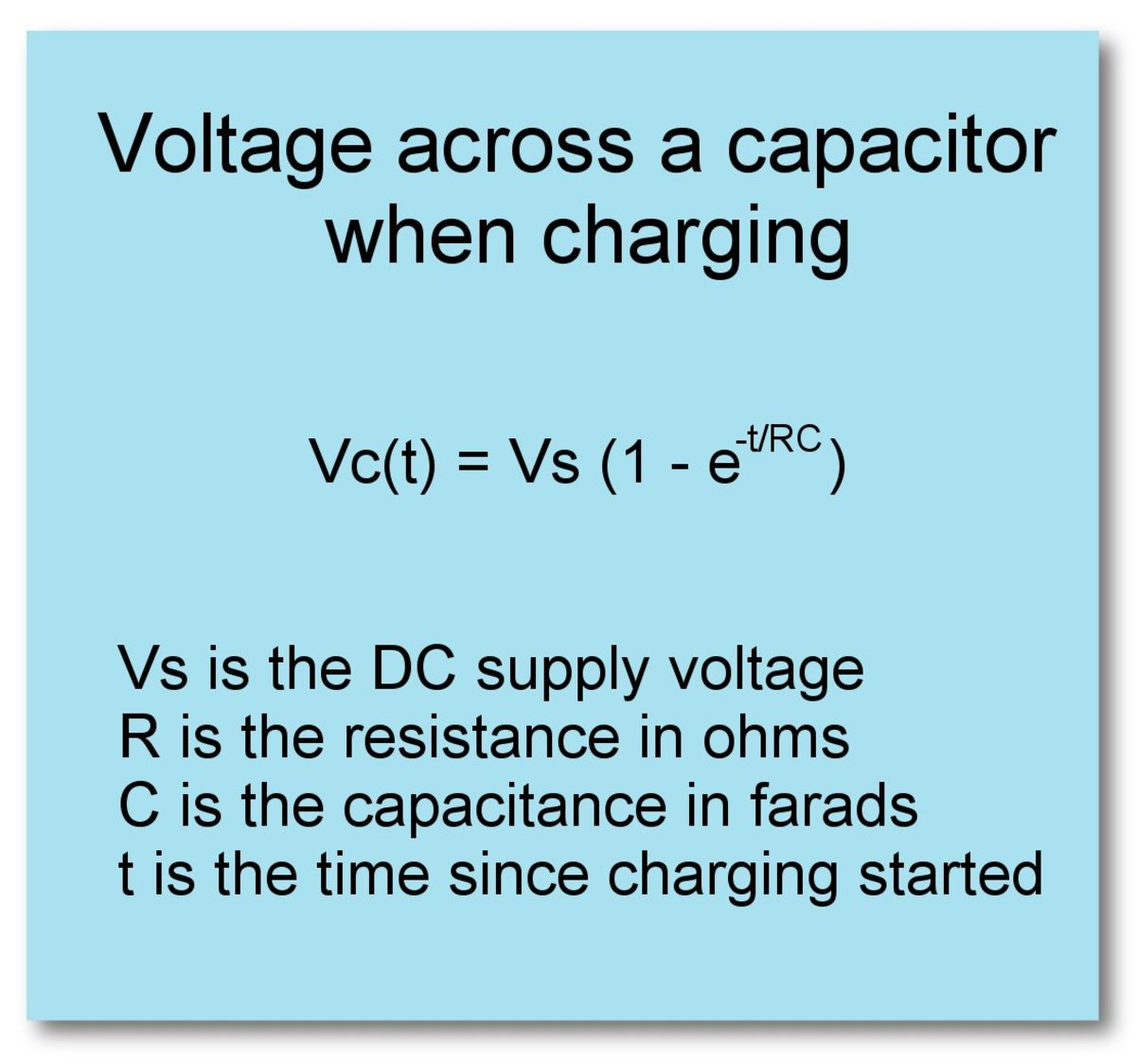Equation for voltage on a capacitor in an RC circuit as the capacitor charges© Eugene Brennan

## Transient Response of an RC Circuit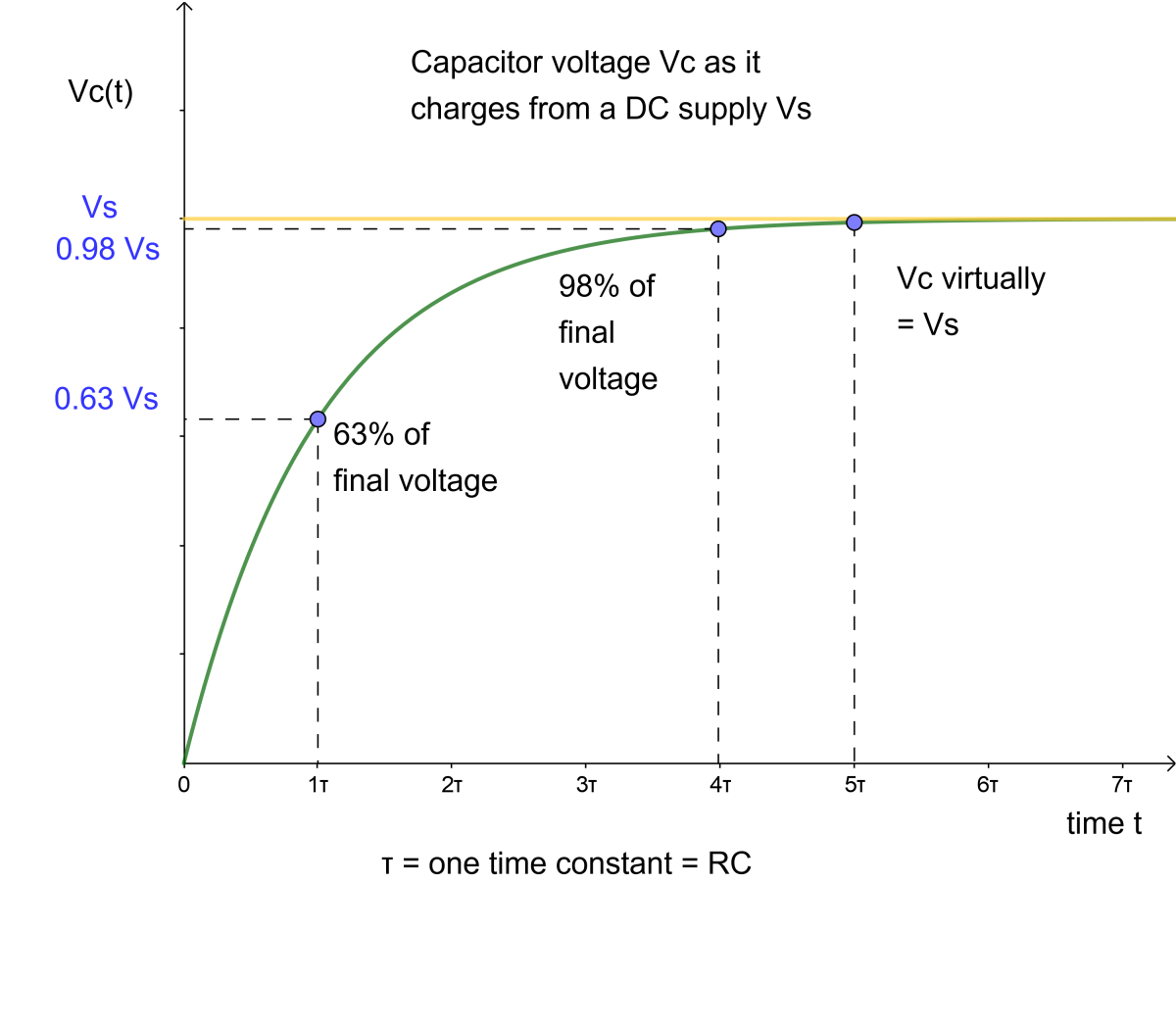Graph of the step response of an RC circuit© Eugene Brennan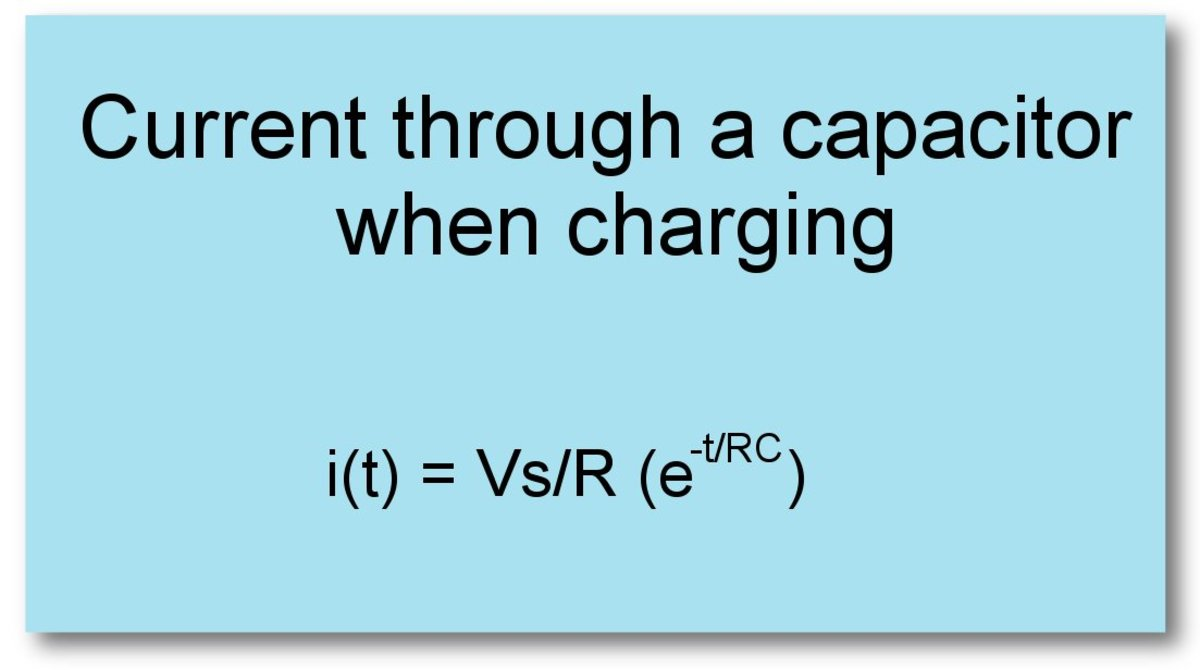Current through a capacitor in an RC circuit during charging© Eugene Brennan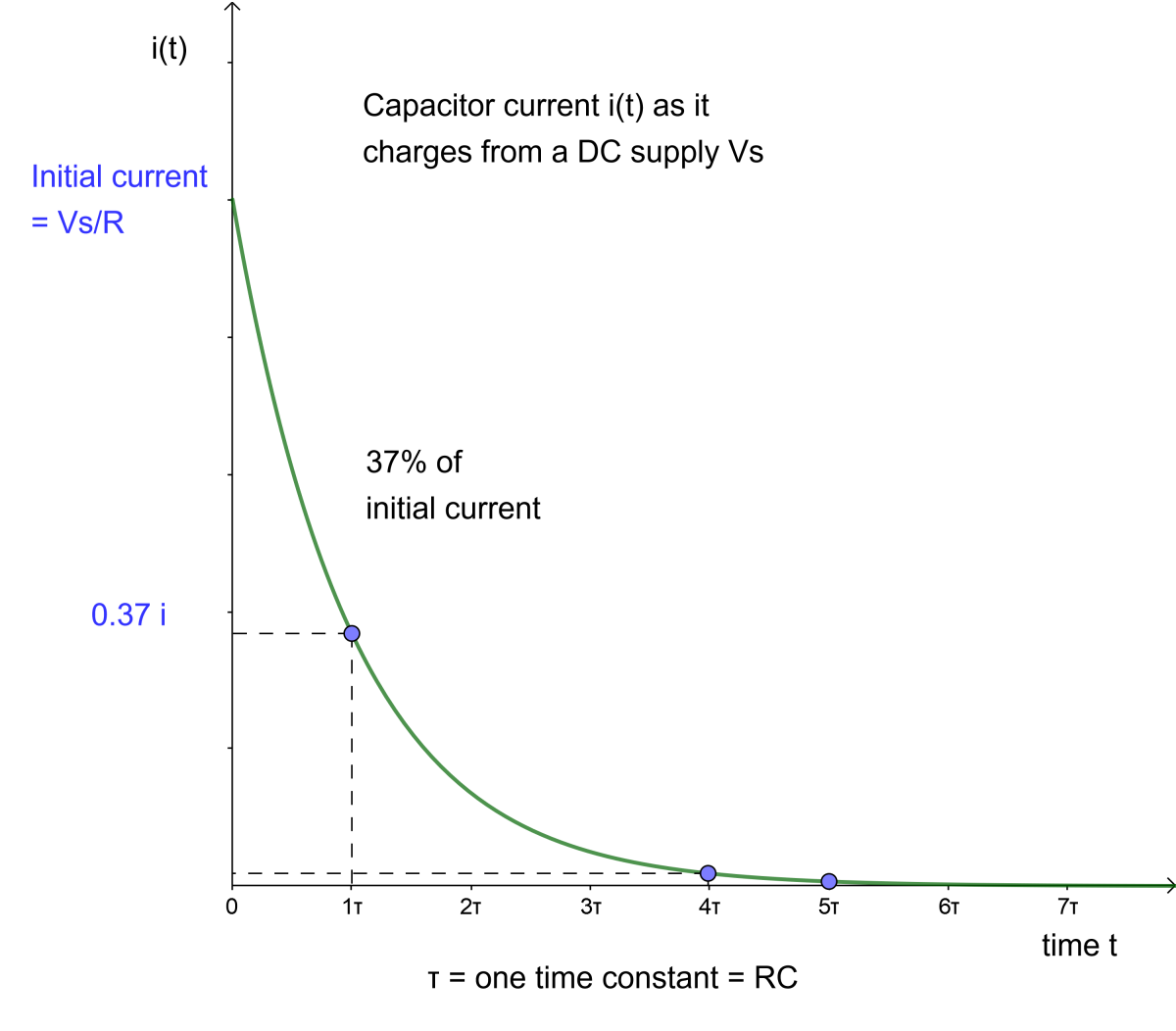Graph of capacitor current for an RC circuit© Eugene Brennan

## Discharge Equations and Curves for an RC Circuit

Once a capacitor is charged, we can replace the supply with a short circuit and investigate what happens to capacitor voltage and current as it discharges. This time current flows out of the capacitor in the reverse direction. In the circuit below, we take KVL around the circuit in a clockwise direction. Since current flows anticlockwise, the potential drop across the resistor is positive. The voltage across the capacitor "points the other way" to the clockwise direction we're taking KVL, so its voltage is negative.

So this gives us the equation:

i(t)R - Vc(t) = 0

Note that we could have taken KVL anti-clockwise around the loop, then the equation would be: Vc(t) - i(t)R = 0 . The important thing is that we assume a current direction first, then stay consistent. So voltage sources "pointing" and more positive in the direction we take KVL are positive and current flowing in the direction we take KVL cause negative PD across resistors.

Again the expression for voltage and current can be found by working out the solution to the differential equation for the circuit.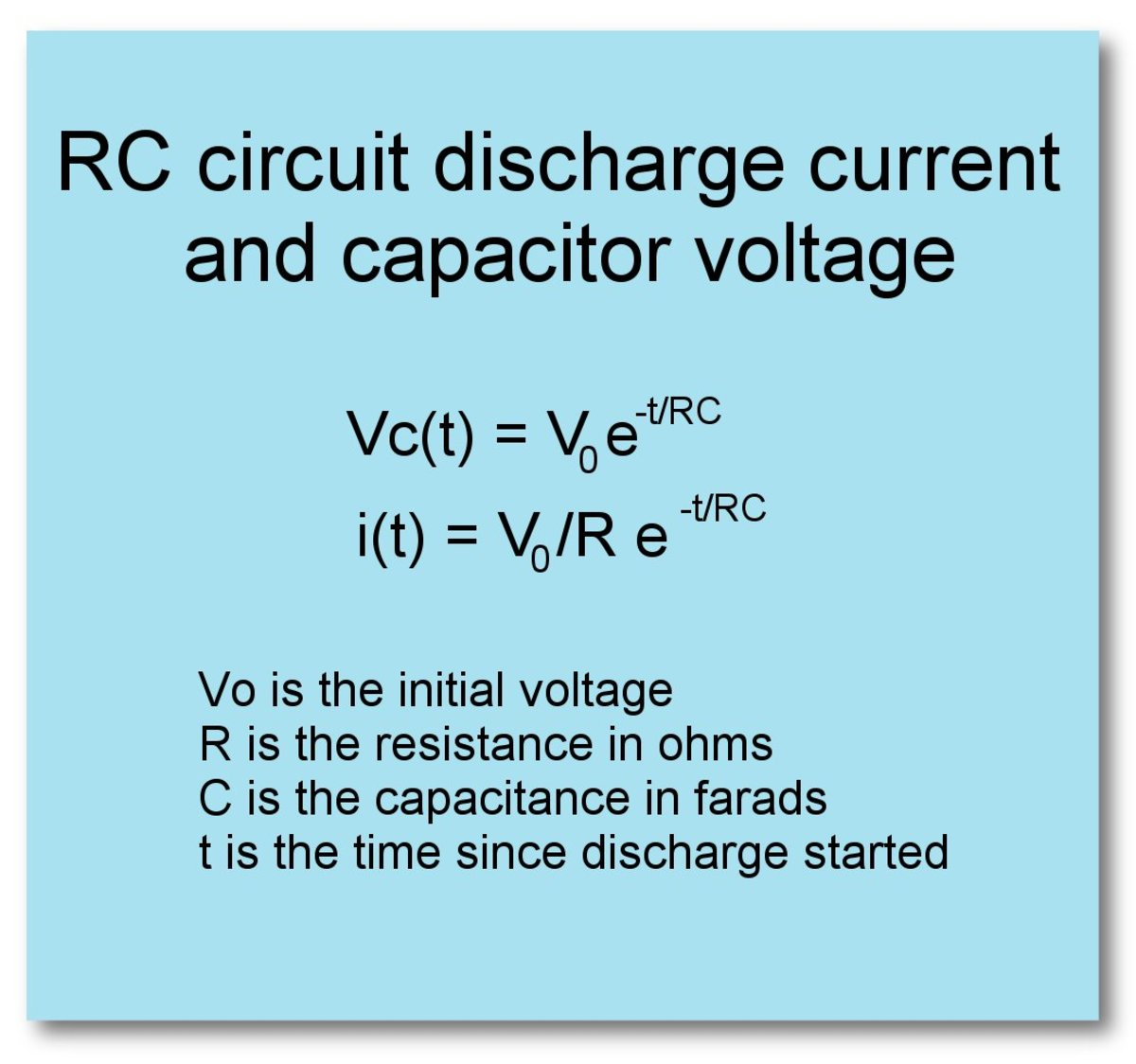Equations for discharge current and voltage for an RC circuit© Eugene Brennan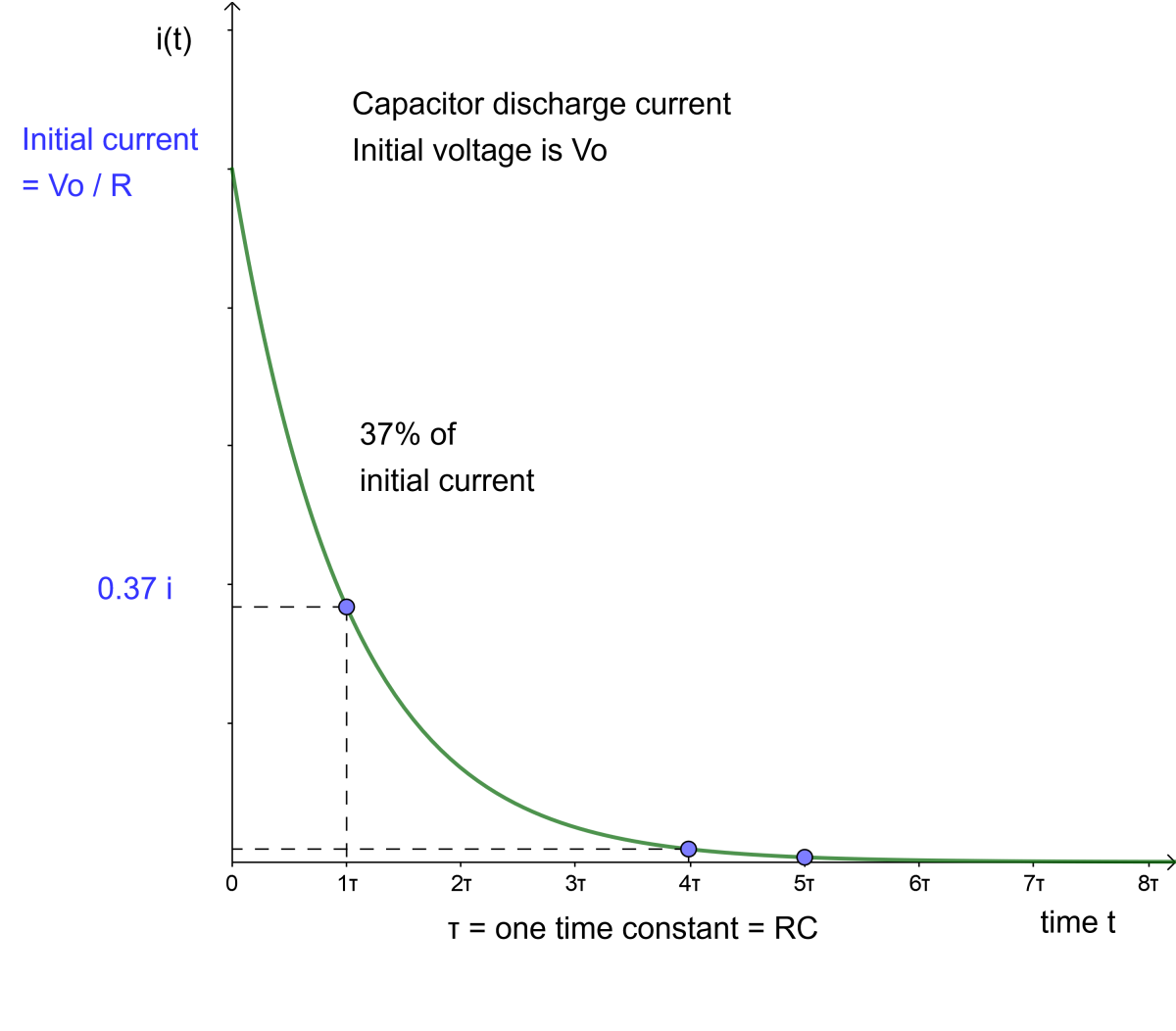Graph of discharge current through a capacitor in an RC circuit© Eugene Brennan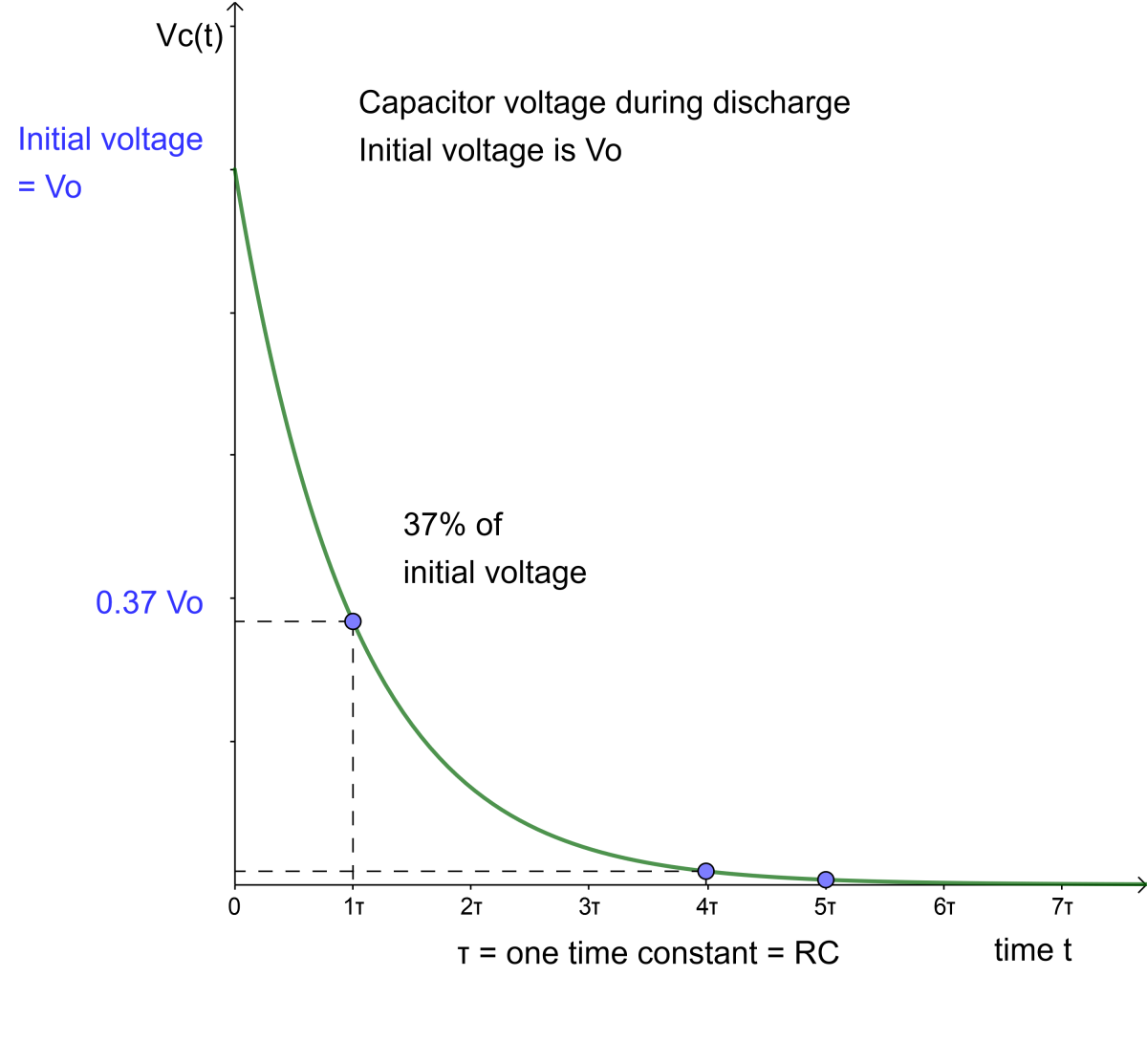Voltage on a capacitor in an RC circuit as it discharges through the resistor R© Eugene Brennan

Example:

An RC circuit is used to produce a delay. It triggers a second circuit when it's output voltage reaches 75% of its final value. If the resistor has a value of 10k (10,000 ohms), and triggering must occur after an elapsed time of 20ms, calculate a suitable value of capacitor.

We know the voltage on the capacitor is Vc(t) = Vs (1 - e-t/RC)

The final voltage is Vs

75% of the final voltage is 0.75 Vs

So triggering of the other circuit occurs when:

Vc(t) = Vs (1 - e-t/RC) = 0.75 Vs

Dividing both sides by Vs and replacing R by 10 k and t by 20ms gives us:

(1 - e-20 x 10^-3/(10^4 x C)) = 0.75

Rearranging

e-20 x 10^-3/(10^4 x C) = 1 - 0.75 = 0.25

Simplifying

e-2 x 10 ^ -7 / C = 0.25

Take the natural log of both sides:

ln(e-2 x 10 ^ -7 / C ) = ln (0.25)

But ln (ea) = a

So:

-2 x 10-7 / C = ln (0.25)

Rearranging:

C = (-2 x 10-7) / ln (0.25)

= 0.144 x 10-6 F or 0.144 μF

## The 555 Timer IC

The 555 timer IC (integrated circuit) is an example of an electronic component that makes use of an RC circuit to set timing. The timer can be used as an astable multivibrator or oscillator and also a one-shot monostable multivibrator (it outputs a single pulse of varying width every time its input is triggered).
The time constant and frequency of the 555 timer are set by varying the values of a resistor and capacitor connected to the discharge and threshold pins.

Datasheet of the 555 timer IC from Texas Instruments.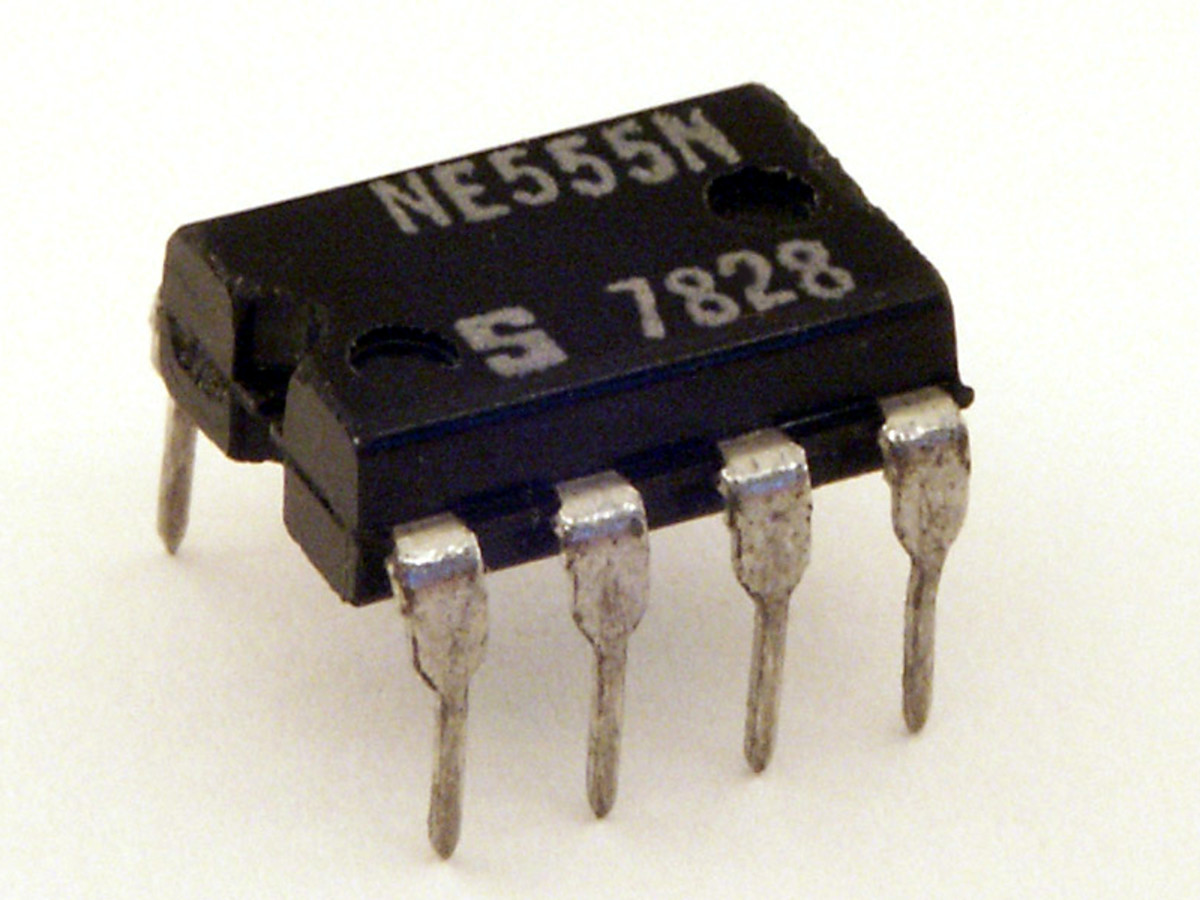555 timer ICStefan506, CC-BY-SA 3.0 via Wikimedia Commons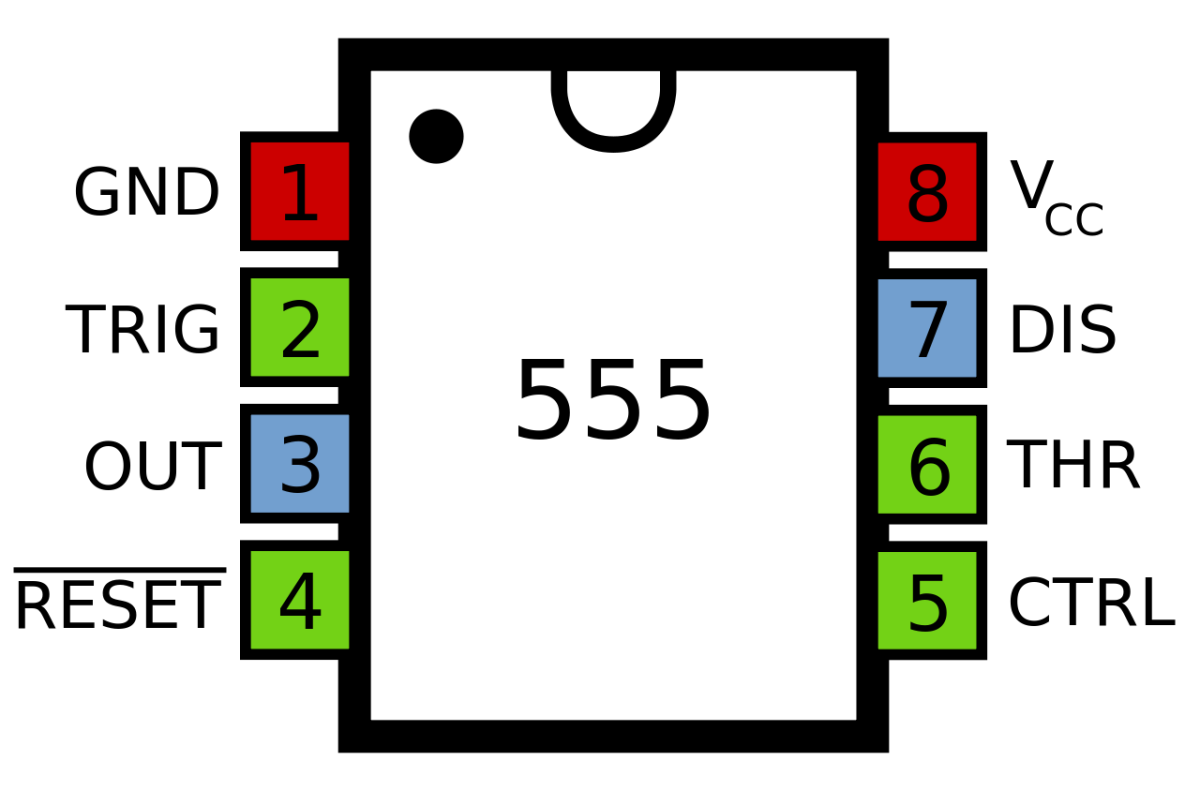Pinout of the 555 timer ICInductiveload, public domain image via Wikipedia Commons

Introductory Circuit Analysis by Robert L Boylestad covers the basics of electricity and circuit theory, with examples of working out currents and voltages in DC circuits and transient analysis. It also covers more advanced topics such as AC theory, magnetic circuits and electrostatics. It's well illustrated and suitable for high school students and also first and second year electric or electronic engineering students. This hardcover 10th edition is available from Amazon with a "good - used" rating. Later editions are also available.